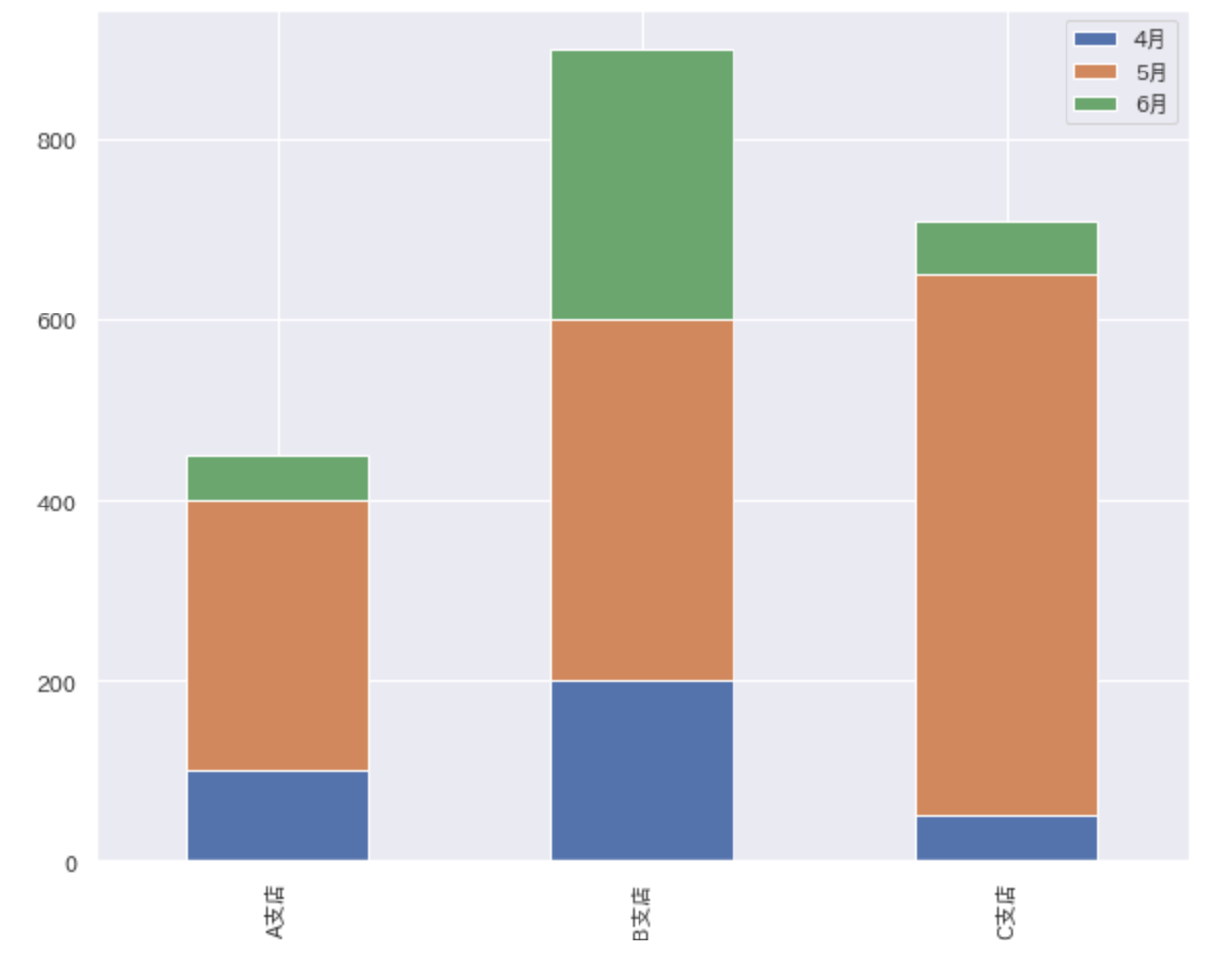# Try drawing a stacked bar graph with matplotlib

## motivation

When drawing a stacked bar graph in Python, I wish I could display the data label like Excel, and when I looked it up, there was no article that could display the data label, and there was no code that I could copy and paste. So, I wrote a code that I can copy and paste when I want to add a data label to the stacked bar graph.

By the way, if you only need to draw a stacked bar graph, please refer to ** Easy to draw a stacked bar graph ** at the end of this page.

## import

Import the following libraries.

``````
import pandas as pd
import numpy as np
import matplotlib.pyplot as plt
import seaborn as sns

sns.set(font='IPAexGothic')

%matplotlib inline
``````

## Creating dummy data

Dummy data created monthly sales data for A, B, and C branches.

``````
dataset = pd.DataFrame([[100, 200, 50], [300, 400, 600], [50, 300, 60]],
columns=['Branch A', 'B branch', 'C branch'],
index=['April', 'May', 'June'])
``````
Branch A B branch C branch
April 100 200 50
May 300 400 600
June 50 300 60

## Draw a bar graph

First, let's draw a simple bar graph. Let's draw a bar graph that totals the monthly sales of each branch. It's good to draw, but with this alone, I don't know how much sales each month. .. .. In such a case, let's use a stacked bar graph!

``````fig, ax = plt.subplots(figsize=(10, 8))
ax.bar(dataset.columns, dataset.sum())
plt.show()
``````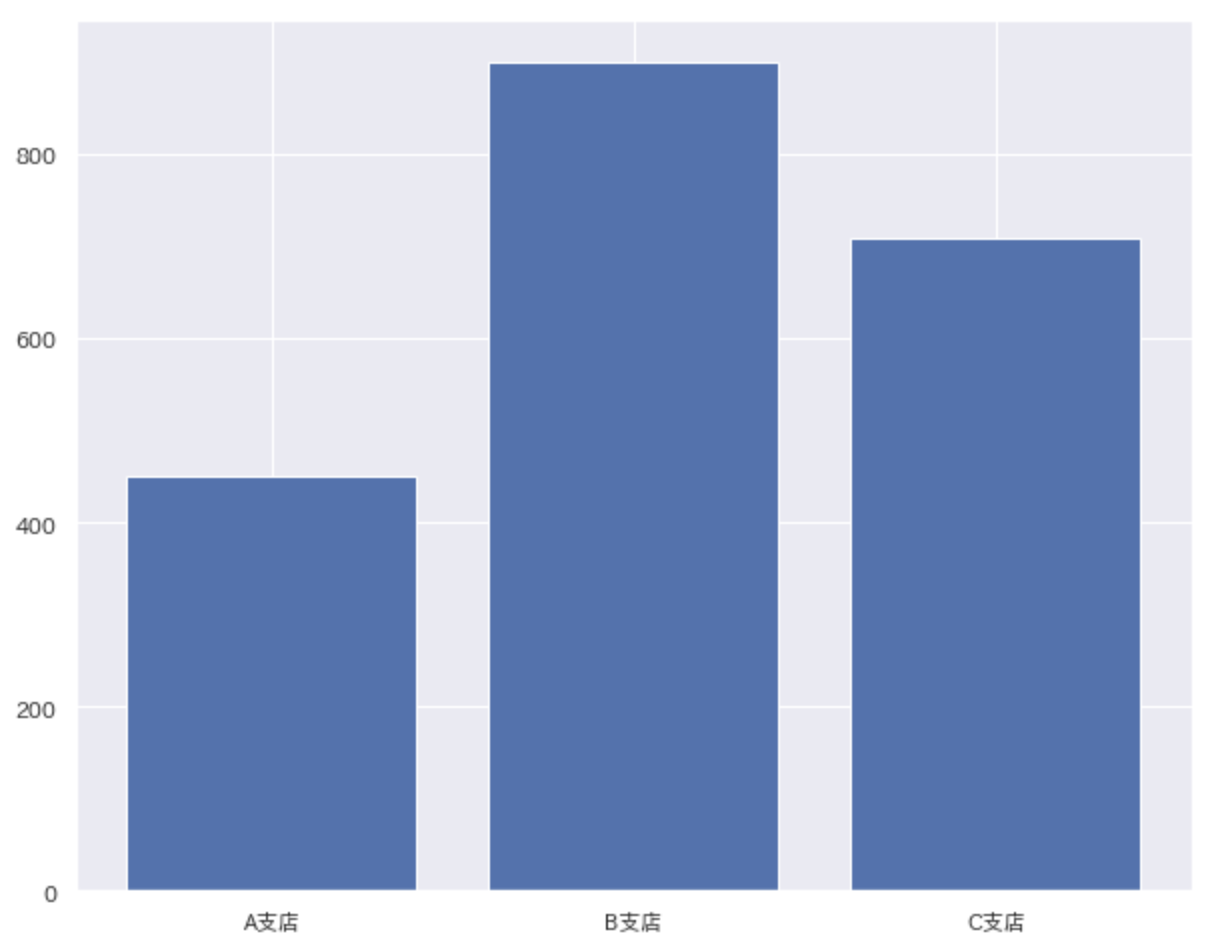## Draw a stacked bar graph

Stacked bar charts use ʻax.bar () `as you would when drawing a bar chart. Specify the y coordinate that is the base of the bar in the argument `bottom` at this time. You now have a stacked bar chart! !! !! After all, I want a data label. Reference site: https://matplotlib.org/3.1.1/api/_as_gen/matplotlib.axes.Axes.bar.html

``````
fig, ax = plt.subplots(figsize=(10, 8))
for i in range(len(dataset)):
ax.bar(dataset.columns, dataset.iloc[i], bottom=dataset.iloc[:i].sum())
ax.set(xlabel='Branch name', ylabel='Sales')
ax.legend(dataset.index)
plt.show()
``````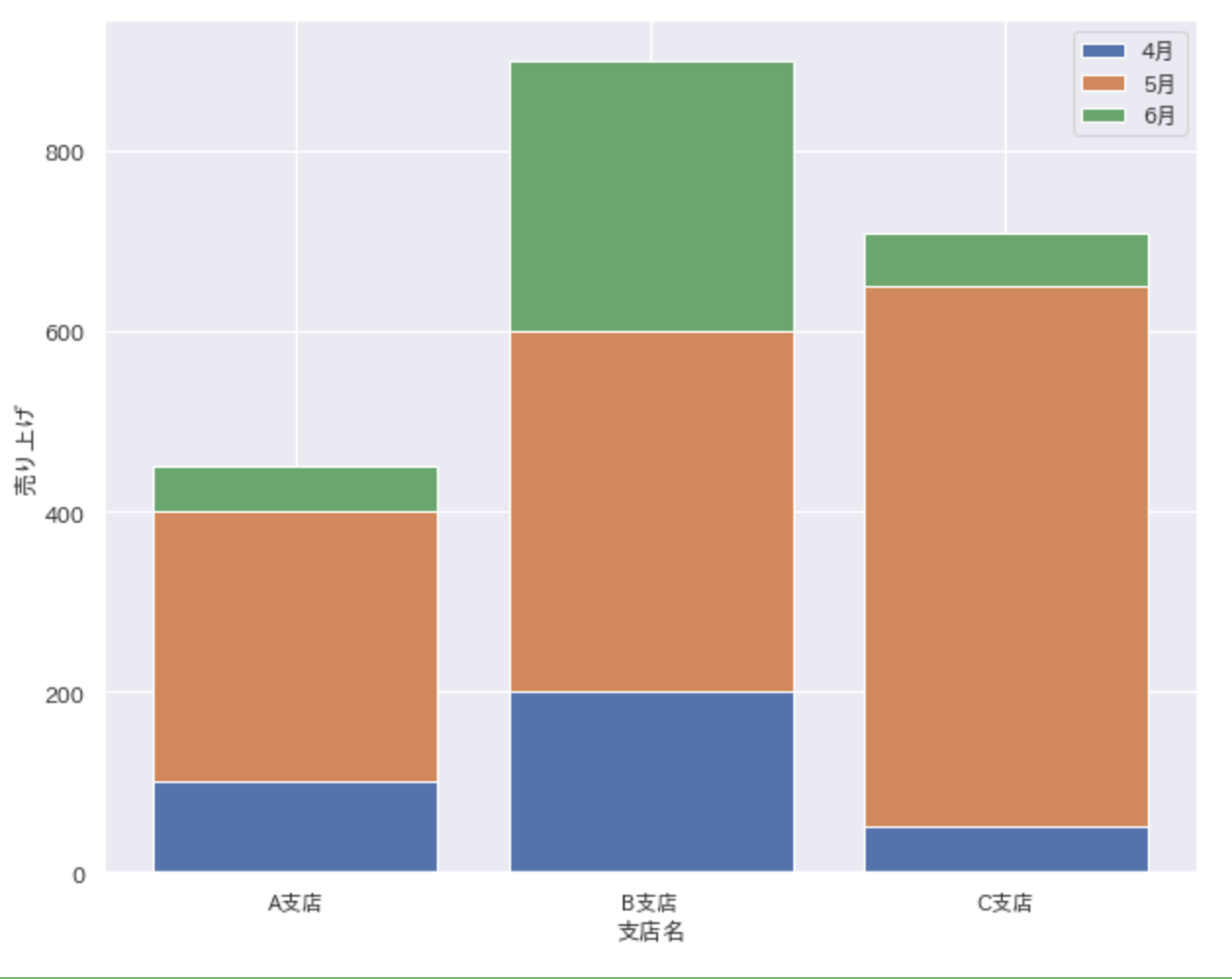## Display data labels in stacked bar charts

The main subject is finally from here. Insert the data label by specifying the x and y coordinates with `plt.text ()`. Then I got a good feeling!

``````
fig, ax = plt.subplots(figsize=(10, 8))
for i in range(len(dataset)):
ax.bar(dataset.columns, dataset.iloc[i], bottom=dataset.iloc[:i].sum())
for j in range(len(dataset.columns)):
plt.text(x=j,
y=dataset.iloc[:i, j].sum() + (dataset.iloc[i, j] / 2),
s=dataset.iloc[i, j],
ha='center',
va='bottom'
)
ax.set(xlabel='Branch name', ylabel='Sales')
ax.legend(dataset.index)
plt.show()
``````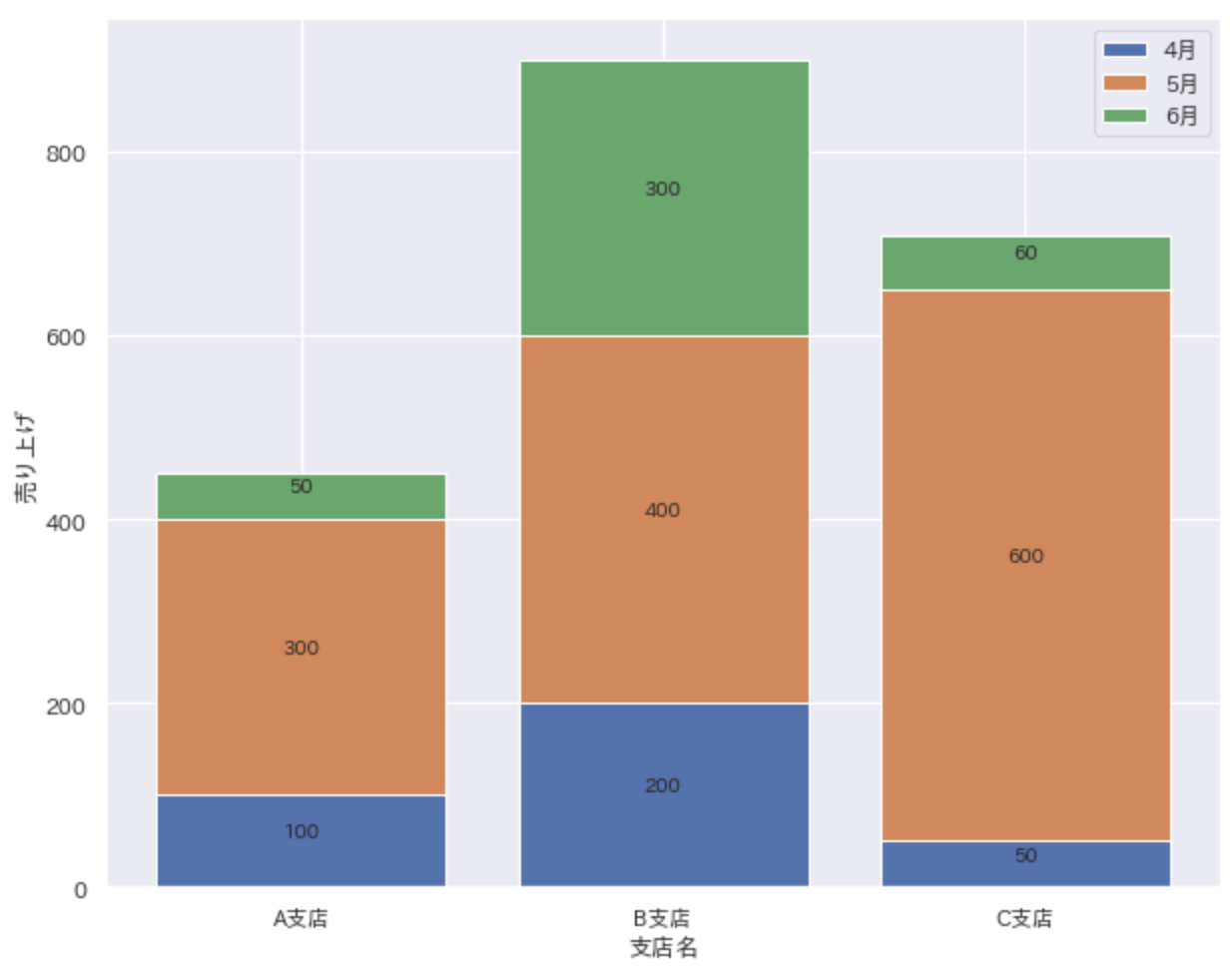## Draw a 100% stacked bar graph

When you use a stacked bar chart, you also want to use a 100% stacked bar chart. First, process the data. The following code converts sales by branch into percentages.

``````
plot_dataset = pd.DataFrame(index = dataset.index)
for col in dataset.columns:
plot_dataset[col] = round(100 * dataset[col] / dataset[col].sum(), 1)
``````
Branch A B branch C branch
April 22.2 22.2 7.0
May 66.7 444. 84.5
June 11.1 33.3 8.5

Draw a 100% stacked bar chart using the converted data. The code is the same as the previous code. (Only variables are changed.)

``````
fig, ax = plt.subplots(figsize=(10, 8))
for i in range(len(plot_dataset)):
ax.bar(plot_dataset.columns,
plot_dataset.iloc[i],
bottom=plot_dataset.iloc[:i].sum()
)
for j in range(len(plot_dataset.columns)):
plt.text(x=j,
y=plot_dataset.iloc[:i, j].sum()+(plot_dataset.iloc[i, j]/2),
s=f'{plot_dataset.iloc[i, j]}%',
ha='center',
va='bottom'
)
ax.set(xlabel='Branch name', ylabel='Sales')
ax.legend(plot_dataset.index)
plt.show()
``````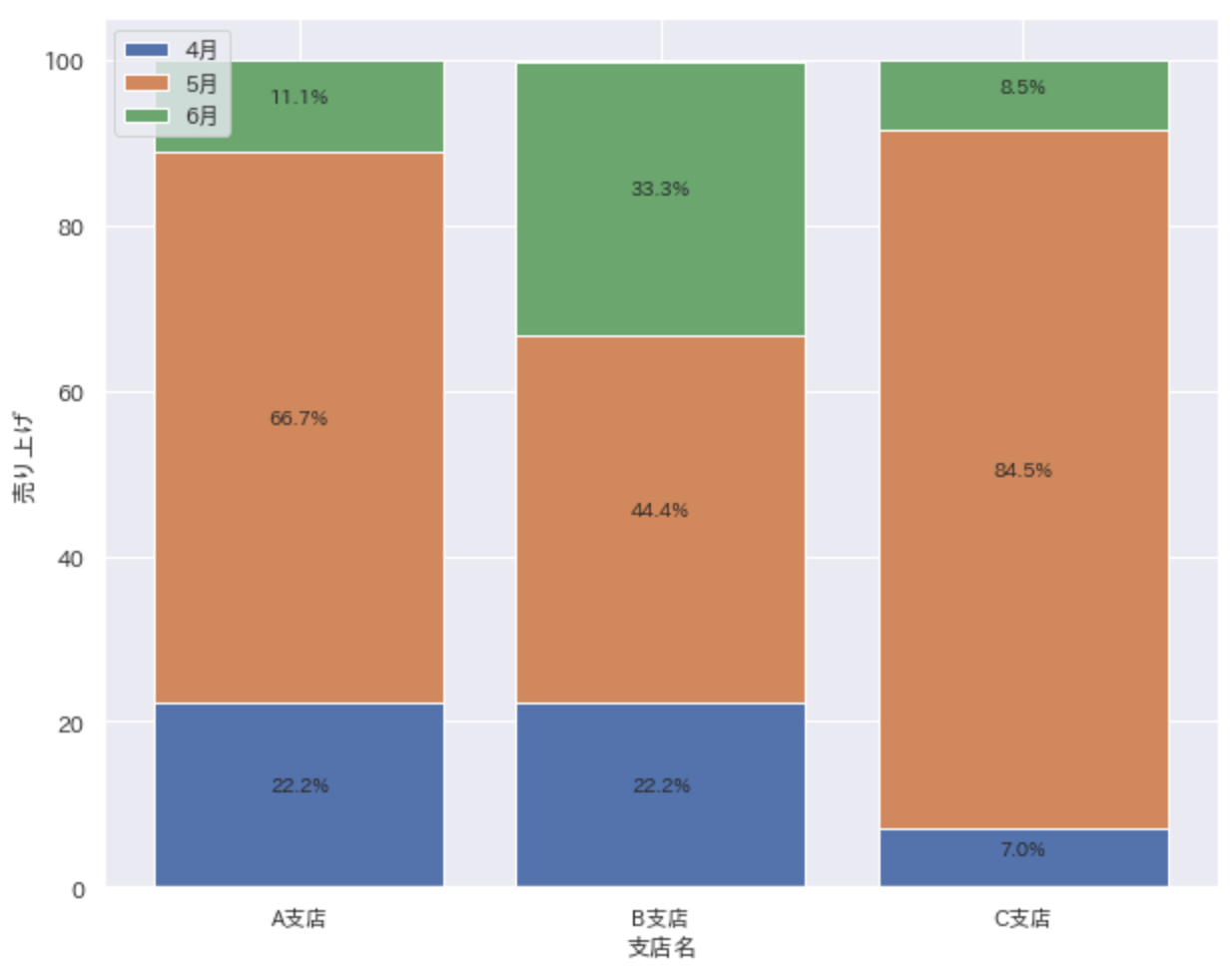## Easily draw stacked bar charts

If you just want to draw a stacked bar graph, you can easily draw it with the following code.

``````fig, ax = plt.subplots(figsize=(10, 8))
dataset.T.plot(kind='bar', stacked=True, ax=ax)
plt.show()
``````